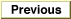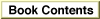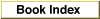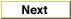# Legacy DocumentImportant: The information in this document is obsolete and should not be used for new development.

Inside Macintosh: QuickDraw GX Environment and Utilities /
Chapter 8 - QuickDraw GX Mathematics / Using QuickDraw GX Mathematics

## Performing Fixed-Point Operations

You can use QuickDraw GX functions to provide operations on `Fixed`, `long`, `fract` and `wide` numbers. The equivalent QuickDraw GX fixed-point functions for functions in the Macintosh Mathematical Utilities is shown in Table 8-2.
Table 8-2 QuickDraw GX and Macintosh Toolbox fixed-point functions
QuickDraw GXMacintosh Mathematical Utilities
`FractDivide``FracDiv`
`FractMultiply`FracMul
`FractSquareRoot``FracSqrt`
`FixedDivide``FixDiv`
`FixedMultiply``FixMul`
`WideMultiply``LongMul`

The Macintosh Mathematical Utilities are described in Inside Macintosh: Operating System Utilities.

Some functions combine multiple functions into a single function to increase calculation speed over that obtained using sequential function calls. For example, the `FractSineCosine` function returns both the sine and cosine of an angle.

Some functions support the use of 64-bit numbers to increase the accuracy of calculations. For example, the `WideAdd` function returns the 64-bit sum of two 64-bit numbers, and the `WideDivide` function returns the quotient of a 64-bit number and a 32-bit number. The `MultiplyDivide` function uses a 64-bit intermediate result to increase accuracy of the calculation and to prevent premature overflow.

The `MultiplyDivide`, `Magnitude`, and `VectorMultiplyDivide` functions are derivatives of other functions. For example, `MultiplyDivide (x, y, z)` is the same as:

```wide temp;
WideDivide (WideMultiply(x, y, &temp), z, 0)
```
The final argument of 0 specifies that the returned number will be rounded with no remainder.

You can use the `Magnitude` function to determine the magnitude (length) of a two-dimensional vector, or the distance between two points on a plane. Figure 8-18 shows the use of function parameters `deltaX` and `deltaY`.

Figure 8-18 Determining the length of a line with the `Magnitude` function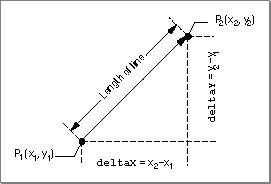Functions that provide arithmetic operations on fixed-point numbers are described in the section "Fixed-Point Operations" beginning on page 8-42. Functions that provide operations on `wide` numbers are described in the section "Operations on wide Numbers" beginning on page 8-49. The `Magnitude` function is described on page 8-45.